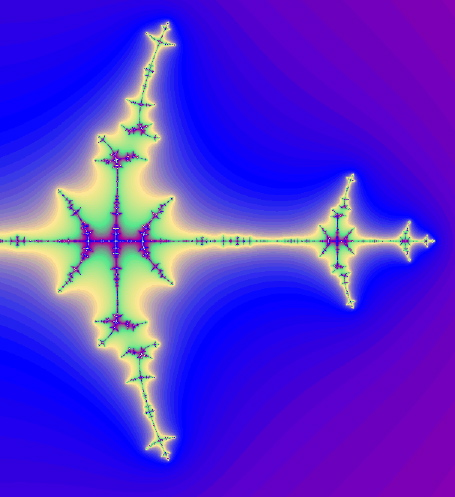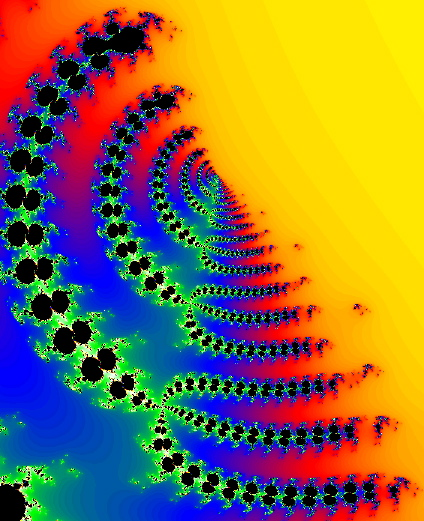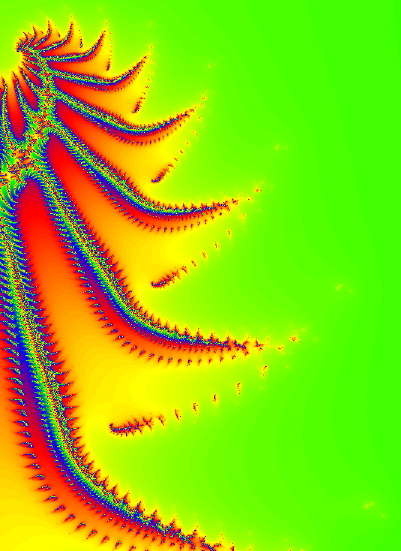Henon Map Dynamical Space Pics: 3

The following images were all created using FractalAsm, and the Henon_Unstable_Manifold definition.

These images show parameterized slices of the unstable manifold of a saddle fixed point of a Henon map, H. Points are drawn black if their forward orbit under H appears to be bounded, or else they are colored according to rate of escape. The saddle fixed point is the rightmost tip of the black.above: (c=-1.4, a=-0.3). These were Henon's original parameters.

below: (c=0.20685+0.483i,a = 0.25+0.6i). We call it "Pitchforks".below: (c=1.01+0.00536, a=.99999). This spiny guy looks like a cantor set.Page 1:Henon unstable manifold pics
Page 2:Henon unstable manifold pics